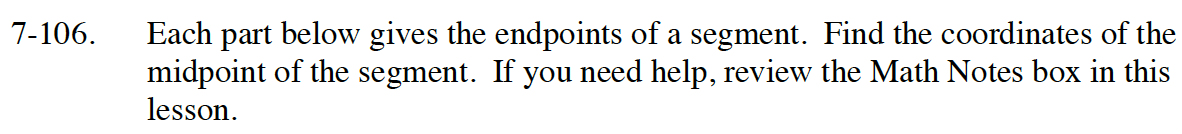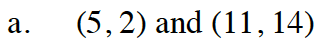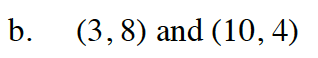### Home > CC4 > Chapter 7 > Lesson 7.2.3 > Problem7-106

7-106.
1. Each part below gives the endpoints of a segment. Find the coordinates of the midpoint of the segment. If you need help, review the Math Notes box in this lesson. Homework Help ✎

1. (5, 2) and (11, 14)

2. (3, 8) and (10, 4)Average the x-coordinates.

$x = \frac{5 + 11}{2} = 8$

Average the y-coordinates.

$y = \frac{2 + 14}{2} = 8$

The midpoint is at (8,8).Follow the same procedures to find the midpoints of the other segment.